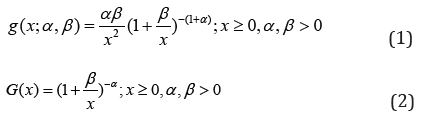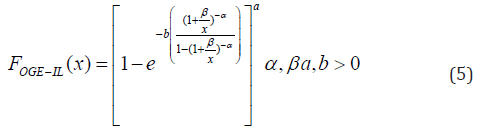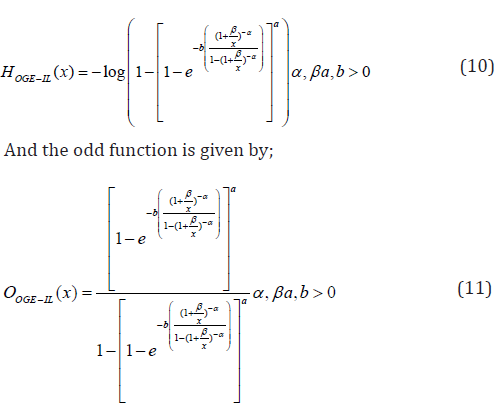Email Us: info@lupinepublishers.comCall Us: +1 (914) 407-6109   57 West 57th Street, 3rd floor, New York - NY 10019, USA

# Lupine Publishers Group

## Lupine Publishers

Submit Manuscript

ISSN: 2644-1381# Modeling Lifetime Data with the Odd Generalized Exponentiated Inverse Lomax Distribution Volume 1 - Issue 3

Obubu Maxwell1*, Nwokike Chukwudike C2, Offorha Bright C3, Olayemi Joshua I4 and Eghwerido Joseph Thomas5

• 1 Department of Statistics, Nnamdi Azikiwe University, Awka, Nigeria
• 2,3Department of Statistics, Abia State University, Uturu, Nigeria
• 4 Department of Mathematical Sciences, Federal University Gusau, Nigeria
• 5Department of Mathematics and Computer Science, Federal University of Petroleum Resources, Effurun, Nigeria

Received: February 22, 2019;   Published: March 11, 2019

*Corresponding author: Obubu Maxwell, Department of Statistics, Nnamdi Azikiwe University, Awka, Nigeria

DOI: 10.32474/CTBB.2019.01.000112

## Abstract

We propose a four parameter compound continuous distribution in this study. Simulation studies was carried out to investigate the behavior of the proposed distribution, from which the maximum likelihood estimates for the true parameters, including the bias and root mean square error (RMSE) were obtained. The proposed model was applied to the strengths of glass fibres dataset and the result compared to other existing distribution.

Keywords: Odd Generalized Exponentiated Family; Inverse Lomax Distribution; Moments; Reliability Function; Hazard Function; Continuous Distribution

## Introduction

The Lomax distribution also called “Pareto type II” is a special case of the generalized beta distribution of the second kind , and can be seen in many application areas, such as actuarial science, economics, biological sciences, engineering, lifetime and reliability modeling and so on . This heavy-duty distribution is considered useful as an alternative distribution to survival problems and lifetesting in engineering and survival analysis . Inverse Lomax distribution is a member of the inverted family of distributions and discovered to be very flexible in analyzing situations with a realized non-monotonic failure rate . The probability density function and cumulative density function of the Inverted Lomax distribution are given by :In literature, a few generalizations of the inverse Lomax exist . The Odd Generalized Exponential family of distribution developed by  having probability density and cumulative distribution function given by;was used to extend the Inverse Lomax distribution. The goal is to derive a continuous compound distribution that would be robust and tractable than the Inverse Lomax distribution. The rest of the article is structured as follows: In section 2, we derive the cumulative distribution function, probability density function, reliability function, odd function, hazard function, reverse hazard function, and cumulative hazard function of the Odd Generalized Exponentiated Inverse Lomax distribution, and present their respective plots for different values of the parameter. In minute details, we establish the structural properties, which include the asymptotic behavior, moments, quantile function, median, Skewness and kurtosis, in section 3. In section 4, we determined the order statistics and the estimate of the unknown parameter using maximum likelihood estimation techniques. Application to two different lifetime dataset and a conclusion is made in section 5 .

## The Odd Generalized Exponentiated Inverse Lomax (OGE-IL) Distribution

A four parameter Odd Generalized Exponentiated Inverse Lomax distribution was investigated in this section. Obtained by substituting equation (2) into (4), the cumulative distribution function of the OGE-IL distribution is given by ;The corresponding probability density function is given by;The reliability function is given by;The failure rate function/hazard function is given by;The cumulative hazard function is given by;## Simulation

In simulation studies using R software, the behavior of the OGE-IL distribution parameters was investigated. Data sets from the OGE-IL distribution were generated with replication number m=1000, random samples were selected in sizes 50, 100, 200, and 300. The simulation was performed with different true parameter values for four different cases. The selected true parameter values are =0.5, =0.5, a=0.5 and b=0.5; =1.0, =1.0, a=1.0 and b=1.0; =2.0, =2.0, a=2.0and b=2.0; =3.0, =3.0, a=3.0and b=3.0 for the first, second, third, and fourth cases, respectively. Maximum likelihood estimates for the true parameters, including the Bias and Root Mean Square Error (RMSE) were obtained. The results of the simulation study are displayed in Tables 1-4 below.

Tables 1-4 clearly indicate that RMSE decreases when the sample size increases for all selected parameter values. In addition, the variations of the predictions are closer to the actual parameter values, with the increase in the sample size decreasing overall bias. Thus, the estimates tend approaches the true parameter value, as the sample size increases.

## Application

The Odd Generalized Exponentiated Inverse Lomax distribution was applied to a real-life dataset and its performance was compared to the Odd Generalized Exponentiated Exponential Distribution (OGE-E) , and the Exponential Exponentiated Distribution. The most suitable selection criteria were based on the values of the Loglikelihood, and, Akaike Information Criterion (AIC),

### Data I: Strengths of Glass Fibres Dataset

The dataset obtained from Smith and Naylor  represents the strengths of 1.5 cm glass fibres, measured at the National Physical Laboratory, England. The observations are as follows;

0.55, 0.93, 1.25, 1.36, 1.49, 1.52, 1.58, 1.61, 1.64, 1.68, 1.73, 1.81, 2.00, 0.74, 1.04, 1.27, 1.39, 1.49, 1.53, 1.59, 1.61, 1.66, 1.68, 1.76, 1.82, 2.01, 0.77, 1.11, 1.28, 1.42, 1.50, 1.54, 1.60, 1.62, 1.66, 1.69, 1.76, 1.84, 2.24, 0.81, 1.13, 1.29, 1.48, 1.50, 1.55, 1.61, 1.62, 1.66, 1.70, 1.77, 1.84, 0.84, 1.24, 1.30, 1.48, 1.51, 1.55, 1.61, 1.63, 1.67, 1.70, 1.78, 1.89.

(Tables 5 & 6) Table 6, the OGE-IL has the highest log-likelihood value and the lowest AIC value; hence, amongst the distributions considered, it is the most appropriate model.

## Conclusion

Using the Odd Generalized Exponential family of distribution, we have successfully extended the Inverse Lomax distribution from a two parameter to a four-parameter distribution. Densities and theoretical characteristics for the proposed OGE-IL distribution have been derived. Simulation studies reveal that the root mean square error decreases for the selected parameter values when the sample size increases. The proposed distribution was applied to a lifetime dataset and compared to other existing distribution which it outperformed based on log-likelihood and AIC values.

Close## Online Submission System

### Submit ManuscriptClick here for Manuscript Sample Templates### Drag and drop files here

or

( For multiple files submission, zip them in a single file to submit. For file zipping software Download )